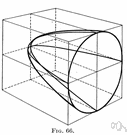# paraboloid

(redirected from Hypar)
Also found in: Thesaurus, Medical, Encyclopedia.

## pa·rab·o·loid

(pə-răb′ə-loid′)
n.
A surface having parabolic sections parallel to a single coordinate axis and elliptic or circular sections perpendicular to that axis.

## paraboloid

(pəˈræbəˌlɔɪd)
n
(Mathematics) a geometric surface whose sections parallel to two coordinate planes are parabolic and whose sections parallel to the third plane are either elliptical or hyperbolic. Equations x2/a2 ± y2/b2 = 2cz

## pa•rab•o•loid

(pəˈræb əˌlɔɪd)

n.
a surface that can be put into a position such that its sections parallel to at least one coordinate plane are parabolas.
[1650–60]
 Noun 1paraboloid - a surface having parabolic sections parallel to a single coordinate axis and elliptic sections perpendicular to that axisplane figure, two-dimensional figure - a two-dimensional shape